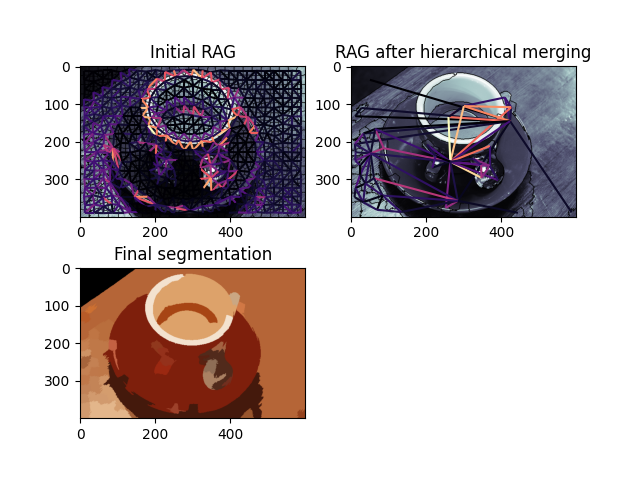# Hierarchical Merging of Region Boundary RAGs#

This example demonstrates how to perform hierarchical merging on region boundary Region Adjacency Graphs (RAGs). Region boundary RAGs can be constructed with the `skimage.graph.rag_boundary()` function. The regions with the lowest edge weights are successively merged until there is no edge with weight less than `thresh`. The hierarchical merging is done through the `skimage.graph.merge_hierarchical()` function. For an example of how to construct region boundary based RAGs, see Region Boundary based RAGs.

•••```from skimage import data, segmentation, filters, color
from skimage import graph
from matplotlib import pyplot as plt

def weight_boundary(graph, src, dst, n):
"""
Handle merging of nodes of a region boundary region adjacency graph.

This function computes the `"weight"` and the count `"count"`
attributes of the edge between `n` and the node formed after
merging `src` and `dst`.

Parameters
----------
graph : RAG
The graph under consideration.
src, dst : int
The vertices in `graph` to be merged.
n : int
A neighbor of `src` or `dst` or both.

Returns
-------
data : dict
A dictionary with the "weight" and "count" attributes to be
assigned for the merged node.

"""
default = {'weight': 0.0, 'count': 0}

count_src = graph[src].get(n, default)['count']
count_dst = graph[dst].get(n, default)['count']

weight_src = graph[src].get(n, default)['weight']
weight_dst = graph[dst].get(n, default)['weight']

count = count_src + count_dst
return {
'count': count,
'weight': (count_src * weight_src + count_dst * weight_dst)/count
}

def merge_boundary(graph, src, dst):
"""Call back called before merging 2 nodes.

In this case we don't need to do any computation here.
"""
pass

img = data.coffee()
edges = filters.sobel(color.rgb2gray(img))
labels = segmentation.slic(img, compactness=30, n_segments=400, start_label=1)
g = graph.rag_boundary(labels, edges)

graph.show_rag(labels, g, img)
plt.title('Initial RAG')

labels2 = graph.merge_hierarchical(labels, g, thresh=0.08, rag_copy=False,
in_place_merge=True,
merge_func=merge_boundary,
weight_func=weight_boundary)

graph.show_rag(labels, g, img)
plt.title('RAG after hierarchical merging')

plt.figure()
out = color.label2rgb(labels2, img, kind='avg', bg_label=0)
plt.imshow(out)
plt.title('Final segmentation')

plt.show()
```

Total running time of the script: ( 0 minutes 1.991 seconds)

Gallery generated by Sphinx-Gallery